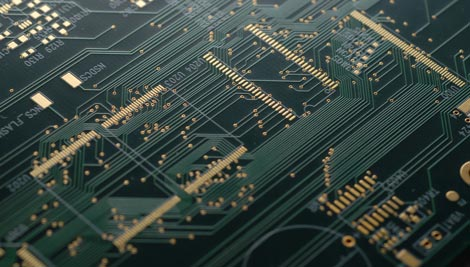Home  > RESOURCES  > PCB Blog  >  What's impedance control in PCB manufacturing

# What's impedance control in PCB manufacturing

2020-06-03

In circuits with resistance, inductance, and capacitance, the impediment to alternating current is called. Impedance is usually expressed by Z, which is a complex number. The real part is called resistance, and the imaginary part is called reactance. Among them, the obstruction effect of capacitors in the circuit on alternating current is called capacitive reactance, and the obstruction effect of inductances in the circuit on alternating current is called. For the inductive reactance, the blocking effect of the capacitor and the inductance on the alternating current in the circuit is collectively called reactance. The unit of impedance pcb is ohm.1) Characteristic impedance

In electronic information products such as computers and wireless communications, the energy transmitted in the PCB circuit is a square wave signal (called pulse pulse) composed of voltage and time. Called characteristic impedance.

(2) Differential impedance

Two identical signal waveforms with opposite polarities input at the driving end are respectively transmitted by two differential lines, and the two differential signals at the receiving end are subtracted. The differential impedance is the impedance Zdiff between the two wires.

(3) Odd-mode impedance

The impedance of one of the two wires to the ground is Zoo, and the impedance of the two wires is the same.

(4) Even mode impedance

The two input signal waveforms with the same polarity at the drive end, the impedance Zcom when the two wires are connected together.

(5) Common mode impedance

The impedance of one of the two wires to ground is Zoe. The impedance of the two wires is the same, which is usually larger than the odd-mode impedance.

PCB circuit board impedance refers to the parameters of resistance and reactance, which hinders the alternating current. In printed circuit board manufacturing, impedance processing is essential.

Chat Online 编辑模式下无法使用
Chat Online inputting...
Please hold on and we will get back to you soon